# Interesting Puzzles

## Puzzle #30 – Who dies if E pushes the stone?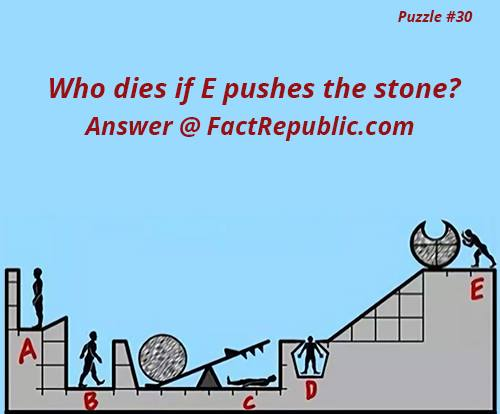E survives.

The ramp is a 3 – 4 – 5 triangle, so the hypotenuse is 5 m (I’m going to say that each space is 1m). The big circle with the hole cut out has a circumference of about 6m, and the cut out is facing the top. Therefore, when the circle rolls down the hill the cut out will be facing downwards when it’s only about halfway down the hill, and will be facing back upwards about when it reaches man D. So, the cutout won’t be in the proper orientation when it reaches him to safely pass. It is to be assumed that he can’t duck in this scenario, so D would die.

The rock hitting D will slow down its velocity enough to not make it miss the right end of the ramp. The rock will come down with enough velocity to crush C, but with not enough force to launch the other rock to A. This is because if you observe closely the left end of the ramp has a little bend on its end which will ensure the rock doesn’t fly the way it is intended to. Mostly, even if the other rock lands on the right end with enough force, the dormant rock will fly up and end on the right end of the ramp as well, making sure C is crushed.

So the only causalities are going to be C and D.

## Puzzle #29 – Tank puzzle 3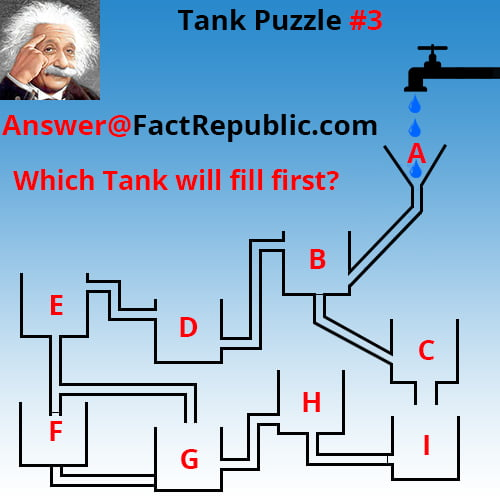## Puzzle #28 – Fruit puzzle 2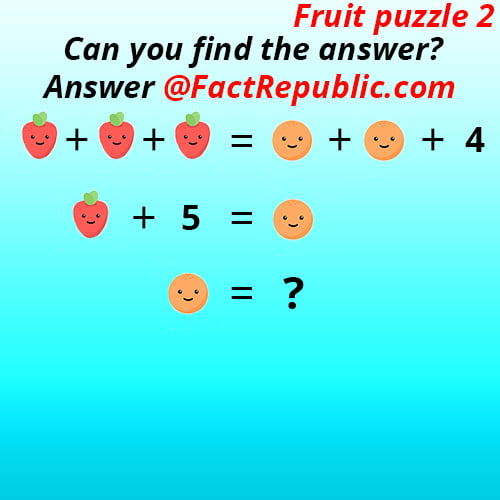Assume the values of apples as x and potatoes as y.
According to the first line of puzzle there are 3 apples and 2 potatoes
Add those we get 3x = 2y + 4 ——> 1 [There are 3 apples so we added “x” 3 times and 2 potatoes so we added “y” 2 times]
In second line of puzzle we get
x + 5 = y ——->2
From the above 2 cases we substitute the value of y in 1st equation, we get
3x = 2 (x + 5) + 4,
3x = 2x + 10 + 4,
3x = 2x + 14,
so x = 14 (The value of apple is 14).
We substitute the value of x in 2nd equation, we get,
14 + 5 = 19 (The value of potato is 19).

## Puzzle #27 – Word puzzle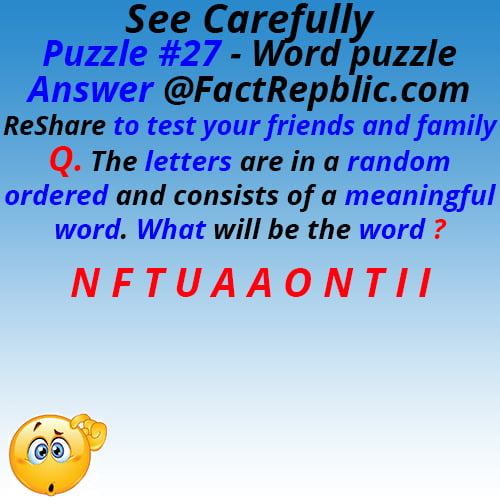## Puzzle #26 – Substitution puzzle3 + 13 x 5 + 9 – 16 / 8 = 3 + 65 + 9 – 2 = 77 – 2 = 75.

## Puzzle #25 – Code puzzle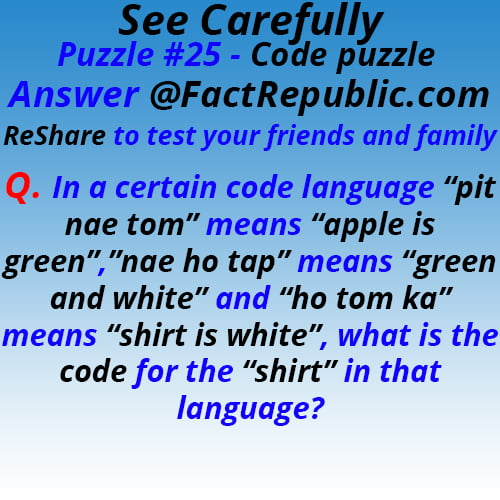According to the three lines of code:
“ka”- shirt,
“nae”- green,
“tom” – is,
“ho”- white,
“pit”- apple,
“tap” – and.

## Puzzle #24 – Time puzzle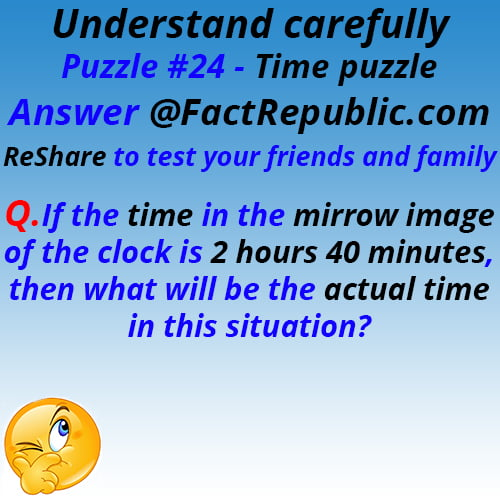The Answer is 10 hours and 20 minutes.

## Puzzle #23 – Interchanging signs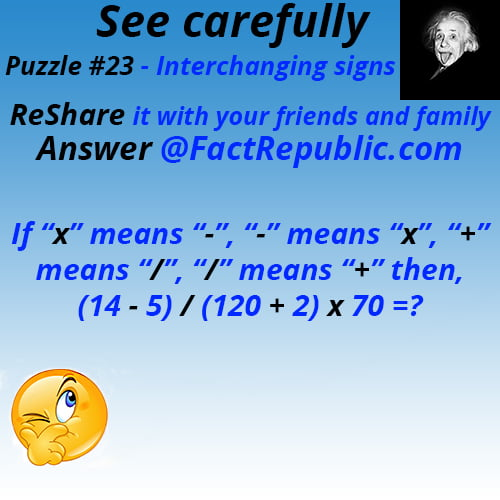According to the question the puzzle will be like this
( 14 x 5 ) + ( 120 /2 ) – 70 = 70 + 60 – 70 = 60.

## Puzzle #22 – Distance Puzzle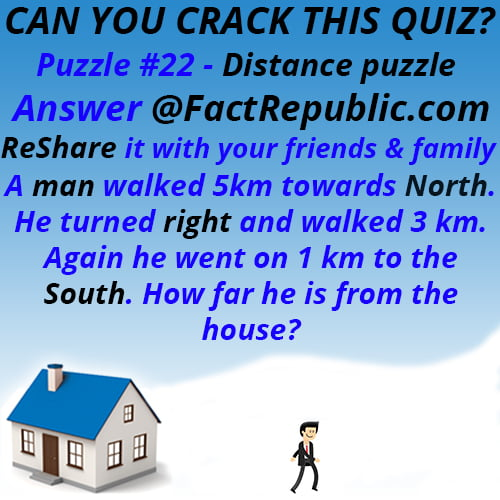## Puzzle #21 – Arithmetic Puzzle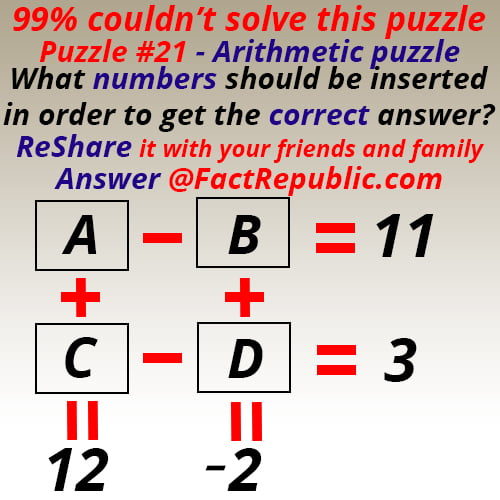A =-25 ,7, 11 , 6 , 12 , 14 , 8, 9
B =-36 , -4 , 0 , -5, -1 , 13, -3 , -2
C = 37 , 5 , 1 , 6 , 0 , -2 , 4 , 3
D = 34 , 2 , -2 , 3 , -3, -5 , 1, 0

1.Naleen

Superb puzzles which are turn around thinking and decisions making ability to a higher level

2. You can’t answer the logical puzzle where there is no way to equate the 2 o’clock clock and 2 5 pointed stars. You can assume that they are worth a value but there is no way to definitively say that this is the correct value as there is no equation to prove them against

•Tasha

If the six pointed star equals 6, the four bladed fan equals 4, and the three bladed fan equals three then logic say a five pointed star is equal to five. If the clock that reads three is equal to three then logic would say the clock that reads two is equal to two.

•Jim Dickinsom

You can not see the answers so it’s pointless. I don’t know whether I am right or wrong

• Thank you, Alan! I agree with your analysis. I have seen similar questions around the Internet. I very seldom see your answer put forth. Although I have tried to point out the fallacy would give way to this:
X + X = 8
X + Y = ?
? = 7 as ‘X’ has four legs and ‘Y’ has three legs, or ? = 9 because of the order of the alphabet. High School and College mathematics teachers beware. There are minions scheming to undo your work!

3.Sam

Which tank fills up first Qs.. Depending on the rate of water that is being poured into A. As if you dump a large amount of water in A at once it will be the first one full. But if the rate of flow is slow then the answer will be F.

•jo

it shows it dripping….

•Me

No need to be a cunt about it

•Mel

Pipe flow is going upstream to F. If water flow is drips L should fill up first

•Ssp

As water is coming to L first, then to F, so L would get fill first.

•Violet

Yes we need to re-evaluate the presence of gravity. If it’s a drip, there’s no pressure to push water into F.

•Christina Freeman

Due to atmospheric pressure working against gravity, there would be significant resistance to the water moving into Tank F. You would find that Tank L would start backing up as well, to be roughly equal to the amount in Tank F. However, this atmospheric pressure is not an issue for water moving from Tank J to Tank I, as the atmospheric pressure in the pipe connecting the two is much less than the forces of gravity and atmospheric pressure in Tank J. This is enough to force water up the pipe to start overflowing into Tank I. It only needs to get higger than the brim of the pipe before gravity works to take it into Tank I. From there the qater will drain into Tank K, long before any overflow into Tank L would cause the levels in either Tank L or Tank F to reach the point of overflowing as described above.

•Mark

Why is it not G?

•Jamie

It isn’t G Mark, because to get to G, the water must first pass through D. And the pipe leading from C to D is blocked. Therefore, you wouldn’t be able to get the water to flow to G.

•RobertAlaska

Because F is not only subject to gravitational force and atmospheric pressure greater than all other buckets, F is also at the lowest height from all others.

•kaylum

in the answer it states that, i will only fill up when j fills up, but before that would happen l would start filling up. ( i think this is because for the water to truvel up a pipe, the previous tank must be full to get the pressure?) so with that in mind, before F can start filling up surely L must be filled up first and the pipe below that eventually feeds tank F as again, the water has to travel upwards to fill tank F?

4.Ronin

@Sam: The water is dripping into tank A from the faucet, according to the illustration.

5.Jim S

In triangle puzzle, as in all Geometry, rather than drawing or coloring each triangle, just letter each intersecting point then just list all by letter (e.g. ABC, ABD, ABE, AFG, AFH, AFE, etc)

6.Christina Freeman

With the water tanks, you forget to take into fluid pressure – the amount of water over a larger surface area in Tank J means that the water would reach higher up the reduced surface area of the pipe to I BEFORE the overflow to L is necessarily reached, and thus I may fill up and drain to K BEFORE the system from L is even reached…

•jess

CF. I won’t fill up because its draining to K as soon as any water enters it due to the drain being at the bottom?

•Anthony

Water fills evenly in a system, so whichever is the lowest will fill first. Assuming that these are all open tanks, as shown in the picture, it will be the only one that fills. Once the water fills F it will begin overflowing and no other tanks will be filled.

•Ron Brown

Er, actually no. The water in the adjacent pipes will always be the same level as in the main tank, assuming that they are all open to atmospheric pressure.
The surface areas of tank and pipes is completely irrelevant. The only pressure in the water is the hydrostatic pressure which is determined by the local depth of the water only, assuming static conditions.
Therefore F will fill first.

•Daniel Burke

Head (height) pressure is independent of surface area. Go back to school, if you ever went.

•Christina Freeman

A larger surface area means more pressure – it also tends to mean a larger volume (given the same depth) which means more gravity affecting it. So how about YOU go back to school?

•Dami

Hi CF. Pressure is inversely proportional to area or volume. Taking that into consideration among other factors, F would get filled up first.

7.Terry

This isn’t Pure math !!!! Stupid

8.jess

Tank I doesn’t need to fill up to drain to K as the drain is at the bottom?

9.Phil Cordingley

In the banana/clock puzzle, I particularly like the incorrect use of the apostrophe. Real genius that!

10.Dano

I don’t see where the input for D is blocked. I say G.

•Sombra

Look closely again. And how is G gonna fill up before F if F is it’s source of water. F has to fill up first before the water could go to G.

•Duane

I agree no where does it show any pipes are plugged. These puzzles dont show us everything

•Kim

D is blocked by C. Definitely F

•Jlg

The outlet from C into D is closed off, it’s not open. Look closely. Therfore, F is the logical conclusion.

11.Dano

Oh. Now I do…..

12.Candice

L because flow to c and D is blocked and flow to H is blocked

13.Gil

i’d like to challenge the creator of this puzzle and say that F is not the right answer according to my theory and how the question is worded…

i know this answer will be the most controversial, but the “Fill Up” answer is A.

if they meant to say “Which Tank Will Spill Over or Fill Up To The Brim”, then yes… F.

but the fill up is only up to the point of what the “tank” can hold. any wall that is built past that “hold” point is just part of the illusion.

•Jo

Tank fill:
1. Depends on your definition of ‘fill’. Is it full when it reaches the pipe and can go no higher or when it reaches the top of the tank?
2. If you define it as the top of the tank, then there are two possible answers – A or F – which depends on whether A drains faster than it fills.

•Craig

So you consider your gas tank “full” when it has hole in it?

•Teq

When something is full, it has reached its maximum capacity. You are arguing “Fill Up” and “Fill Up To The Brim”? Come on, that’s like arguing “Slap Me” and “Slap Me In The Face”, you know exactly what is meant. How can A reach its maximum capacity first? As it is in the process of “FILLING UP” (please note, it is NOT full at this point) it starts feeding tank B.

No need to get technical with wording… It’s Tank F Gil.

14.David imright

I think a would fill up first , to its maximum capacity. You didn’t say fill up to the top !!!

•David imright

“A” aaaaaaaaaaaaaaaa

•Teq

So if A has filled to its “Maximum Capacity” as you say, what would we call it once all the other tanks are filled, then A finally fills to the brim? Would this mean A has filled to more than its Maximum Capacity? No Such.

15.Paul

“share to see you’re friends” not such genius here.

16.Jeremie Perry

On the puzzle of: Which Tank will fill up first? I believe the answer is incorrect. Why Would it not be K? I the J tank once it’s starts to fill, once it passes the first tube it will automatically start to fill the tube as well and slow the process of filling the tank. By the time the tank is filled the first tube will have already started to drain into I which is draining into K and filling K. Once K is about 1/8-1/4 full, F should have started to fill.(that is a lot of water to fill the tube before it Starts to Fill F)

17.Jase

L. Water isnt going to magically fill F when its fed from the bottom. Which means no matter how you set it up L would have to be full before F can be. You’re pushing water up into a container using gravity. If you had two identical containers that are connected at the bottom and started filling one, the displaced water would fill both and the water will level out. They both couldnt be full at the same time unless the one being filled is full first. Jeez its not that hard. Bad fail here

18.TPaull

How does F fill up when it doesn’t even have an access pipe for the water to reach it????

19.Mel

I disagree with the answer to which tank will fill up first. Since the illustration shows water flow as “drips” Tank L would be fill up first because the pipe leading to tank F is going upstream. Not enough water pressure to fill tank F faster than Tank L. Correct me if I’m wrong.

20.PhunniPhan

“Which Tank Fills Up First,” is wrong. But thank you for putting the wrong answer there. I can tell which students are cheating and looking stuff up on their phones instead of applying what I’ve tried to teach in class. Yes, I’m a Physics Teacher. And yes, the correct answer is “K.” But please don’t change it – gives me a teaching moment about cheating….just saying.

•admin

Then you are a bad physics teacher. How will I fill up before L?

•XM

Tank I cannot fill up anything until J is almost full since water will not travel upwards, and for water to reach the top of linking pipe (i and j), then it has to rise at the same level as j. Therefore k is the wrong answer!!!

•Brian Anderson

J only has to fill up a 1/3 before the weight shoots it up the left tube. K wins. Website, deduct a point please

21.Luis brindas

I would like to challenge the creator of the tanks filling up. I think the proper answer is k due to gravity mass. It would push it self threw I from j due to weight of water. Then it would slowly drip into k.

•Brian Anderson

I agree. K first. J only had to fill up a 1/3 before the weight shoots it up the left tube.

22.Glenn

You guys are a bunch of ……smart people…lol. The answer “is” F. The water will travel down from A into B then into C it can’t go into D because the path is blocked (notice the line blocking the fill point) from there it spills into J filling up about halfway then spilling into L where it maintains the level in J while filling the pipe connecting L to F (H is Blocked as is D) when full the water will begin filling F. F will fill and begin to over flow while L will fill to just below the fill pipe. J will never fill I. Water will always level itself if not forced and this is an example of gravity not force.

23. If the inflow on the water puzzle is faster than the outflow, tank A would fill up first. One could assume, by the slow drip in the image, inflow was slow, but the puzzle did not specify.
If one wanted to get real anal, it could be argued we do not know how long the water will run for… but that would be silly ;p

•Cohen Erasmus

Lol saw this comment afterwards #GreatMinds

24.Cohen Erasmus

Why do I think A will fill up first like if the amount of water flowing from the Tap is greater then the amount of the leak a will fill up first regardless of the leak…
By the time water gets to F …. A will be filled long time…

25.Cohen Erasmus

Eish It’s L that will fill if it is dripping …. into and not flowing…..

Coz the water can’t flow up into F

26.David Wallace

There’s a problem with the Matchstick 508 problem. By moving two matches from the 0, you can form 9E8 which equals 900,000,000. I’m not claiming that this is the highest number, but I am claiming it’s higher than the given solution. Perhaps they didn’t expect other number notations.

27.arana

[(9×6)+3]-13=44

Row 1:2*(column number(2))=4 – value in first column(1)=3
Row 2:5*(column number(2))=10 – value in first column(4)=6
Row 3:8*(column number(2))=16 – value in first column(7)=9
Row 4:12*(column number(2))=24 – value in first column(11)=13
Row 5:30*(column number(2))=60 – value in first column(11)=42

Formula works for all the previous results per row, so it should be valid also.

•arana

errata :
seeing puzzle number 9 and your solution, I thought it differently and came up with another that I guess would be also correct maybe you should include it in the answer.

[(9×6)+3]-13=44

Row 1:2*(column number(2))=4 – value in first column(1)=3
Row 2:5*(column number(2))=10 – value in first column(4)=6
Row 3:8*(column number(2))=16 – value in first column(7)=9
Row 4:12*(column number(2))=24 – value in first column(11)=13
Row 5:30*(column number(2))=60 – value in first column(18)=42

Formula works for all the previous results per row, so it should be valid also.

28.Soni

In a real matchstick game situation, the sticks are laid on a table, or such, and people sit around. People in the opposite read the number as 805 instead of 508. from this angle, the answer will be 81151 (ordinary number) or 8E9 (scientific)

29.Noah

The correct answers on most of these puzzles require making assumptions that are not provided by the problem. You could just as easily choose to make different assumptions and therefore get different answers.

•Norm

You are totally correct, Noah! There is not one correct answer to a lot of these “puzzles.” Take the Logical Puzzle. Answer 1- could be 67 (Order of Operations – PEMDAS). Answer 2 – could be 38. Answer 3 could be 35. And the one I like best is Answer 4 – there is no answer because the pics are different in the final line and, therefore, have no value because we weren’t given any way to reference what the new pics should equal. Therefore, there is no way to answer this problem.

30.Ernesto Verano

Matchsticks 508 puzzle. You can take off 2 sticks from zero and put it before number 5 to make it 15118 then reverse the matchsticks making it 81151 as the highest possible answer.

31.Peter

The one with the match sticks. 5118^11. This way the dwarfed numbers are raised and become an exponent.

32.Parzival

The answer to Puzzle #66 is not 60 or 12 it is actually 1 because any number multiplied by zero is going to be zero, its basic math.

•Noah

The order of operations, which you have neglected, is also “basic math.”

33.Noah

#58 is obviously wrong. 5+8=13. The fact that the addition on the previous lines was incorrect is irrelevant.

•Dean

Puzzle #58’s answer is 45 as well.
Because you change the + to a x and then add 1 to the number after the x.
Before: 1+4=5, 2+5=12, 3+6=21 and 5+8=?
After: 1×5=5, 2×6=12, 3×7=21 and 5×9=?=45

34.john sylvester

Puzzle #69. Two penguins in an overlay does not, by definition, mean 2X one penguin. This is especially true when the X symbol is denoted in the equation to indicate a multiplier. Stating the overlayed penguin and an overlayed cow (again ‘especially’, when one is different) are 2X without including the X presumes an embedded ruleset of real numbers. This is recited multiplication table pedantry. The white cow could as easily be presumed to be the negative of the other cow on the same numberline using that ruleset. Equally, even without the different inks on the cows, the total display size of each icon would be a perceived numerical value at the genius level, thus making measurements or calculations of their square space or pixels required. The person developing the puzzle was not a genius nor schooled in communications.

35.sgtstan

5 – 7 = -2

•CAC

That’s what I got (also 5+1 ≠ 2)

•nope

5+7=2
9-2=2

move the match from the + to the top right space of the 5.

36.sgtstan

Problem #24 – (Just to be nit-picky) If the mirror image reads 2:40, then the little hand should point between the indicators for 2 and 3 on the clock face. The reverse of this would place the little hand between the indicators for 9 and 10. The answer would then be 9:20, not 10:20.

37.sgtstan

This is an interesting page of comments! Could somebody explain the posted answer to Problem #66? Having the two top rows of 1’s with plus signs between them and no further information, I see them as nothing but a distraction. The only solvable problem is the bottom row’s equation, the answer being 2.

38.Angelica

#66 said to multiply the results before the times 0 by 0 so that would make everything you did there worthless so the ending result would be 0

39.Madhavan

(1+1+1+1+1)
(1+1+1+1+1)
(1+1*0+1)
=5×5×2

•noah

Where do you get the idea that you’re supposed to multipply.

1+1+1+1+1
1+1+1+1+1
1+1×0+1=?

Assuming we’re solving for “?”, then the answer is 2. The first two lines are red herrings.

• =1+1+1+1+11+1+1+1+11+(1*0)+1
=4+11+3+11+0+1
=30

40.noah

The “corrected” answer to #64 is still wrong.

The question mark is asking for the answer to 1+1×0+1. The answer to that, and this question, is 2.

The idea that the two lines have to connect makes no sense. It’s a line break. There is no 11 and the first two lines are red herrings.

41.Norm

Puzzle #62 the first tea cup to fill first is 4 not five.

42.guest

#52 is questionable… the elephant isn’t “hidden” Also does anyone see the ant?

43.Preeji

Ya I got the 2nd once answer it is 225

44.Vijay

Puzzle 54 ans pls

•Aqati

Bananas clock shapes puzzle/equation

*This is long, so scroll down for the answer, then read through to see how it is solved if you’re curious.

This one truly boggles/frustrates 99.99% of people, because it is probably the most complicated picture/math puzzle out there. This one has many layers, and attention to detail is key. I will show you one of the primary answers people give, then explain to you the failed logic that stems from missing one key component. BTW, whoever created this is truly an evil genius.

First, let’s go through the first line. On the first line, we have 3 objects. Each one we will call a “shape group”. I will tell you up front, the number of sides for each shape group is important. Solving for a single shape group, we have:

shape group + shape group + shape group = 45

We have 3 shape groups, so we re-write it:

3 shape groups = 45
one shape group = 45 / 3
one shape group = 15

Since the number of sides is also important later, let’s count the sides now. There’s a square (4 sides), a pentagon (5 sides), and a hexagon (6 sides). Solving for a single side, we have the equation:

4 sides + 5 sides + 6 sides = 15 sides
15 sides = 15
one side = 15 / 15
one side = 1

So each side in a shape group is 1.

Moving on, we have a bunch of bananas, another bunch of bananas, and a shape group. Up front, the number of bananas matters, and there are four bananas in each bunch. The shape group has 15 sides. Solving for a single banana, we have:

banana bunch + banana bunch + shape group = 23
banana bunch = 4 bananas
shape group = 15 sides
4 bananas + 4 bananas + 15 sides = 23
one side = 1
1 x 15 = 15
4 bananas + 4 bananas + 15 = 23
subtract 15 from both sides
4 bananas + 4 bananas + 15 – 15 = 23 – 15
4 bananas + 4 bananas = 8
4 bananas + 4 bananas = 8 bananas
8 bananas = 8
one banana = 8 / 8
one banana = 1

So each banana is 1.

Next, we have a bunch of bananas and two clocks. As always, paying attention to detail is key. Up front, each position of the hour hand, specifically what hour the number points to, is key. Each clock points to 3, so each clock is 3 hours. Solving for a single hour, we have:

banana bunch + clock + clock = 10
4 bananas in the bunch
1 banana = 1
4 x 1 = 4
4 + clock + clock = 10
clock points to 3 = 3 hours
4 + 3 hours + 3 hours = 10
subtract 4 from each side
4 + 3 hours + 3 hour – 4 = 10 – 4
3 hours + 3 hours = 6
3 hours + 3 hour = 6 hours
6 hours = 6
one hour = 6 / 6
one hour = 1

So each hour on the clock is 1.

Finally! We have all the values, so now we can solve. But wait! The shapes are different! Instead of the clock pointing at 3, it’s pointing at 2! Instead of two bunches of 4 bananas, each bunch has 3! The shape group is missing a square! How are we going to solve this? Getting frustrated yet?

Fortunately, we did all the leg work:

one hour indicated on the clock = 1
one banana = 1
one side of the shape group = 1

Therefore:

2 o’clock = 2 hours indicated = 2
3 bananas = 3
pentagon = 5 sides
hexagon = 6 sides
5 sides + 6 sides = 11 sides = 11

Substituting the numbers, the equation becomes:

2 + 3 + 3 x 11 = ?

So let’s solve!

2 + 3 = 5
5 + 3 = 8
8 x 11 = 88

Wait, something seems off. If it does to you, that’s because it is wrong. What’s wrong? Well, we did all that work, and forgot one of the most basic rules of math: PEMDAS (or BODMAS).
PEMDAS stands for:

Parenthesis
Exponents
Multiplication
Division
Subtraction

It is the order of operations, the order in which math is done. Without this order, equations wouldn’t work. If they didn’t work, we wouldn’t have physics equations, calculus, geometry equations. Without those, planes couldn’t fly, cars couldn’t drive, computers couldn’t work. Heck, these rules are based on an observance of the universe, so our universe wouldn’t even exist without it! It is proof that absolutes exist, and since everything you do in life involves, at a minimum, physics, it is part of your life every second of every day.

So how does it work? Every equation we look at, we follow PEMDAS. First we do all equations in parenthesis. Then we figure out the exponent of a number. Now here is where people seem to forget one of the basic rules of PEMDAS. They think it’s multiplication THEN division. That is incorrect, it is multiplication OR division, specifically whichever comes first (left to right). The same for addition and subtraction, it’s addition OR subtraction, whichever comes first (left to right). There’s more to it that this, but I won’t go into that.

Using PEMDAS, let’s solve:

2 + 3 + 3 x 11 = ?
No parenthesis/exponents, so multiplication first
3 x 11 = 33
33 + 2 = 35
35 + 3 = 38

Yesss!!! Got the answer, it’s 38!

No, it isn’t. Perhaps now you’re frustrated, or even perplexed. There is a component that the VAST majority misses. Before I get to that, I will give some other answers that people get that are incorrect.

3 + 4 + 4 x 15

People will not pay attention. They will say that the shape group is always 15, that the clock is always 3, and that the bananas are always 4. This makes absolutely no sense and has zero logic, especially when faced with everything explained above. If they do this last equation with no PEMDAS, they will get 165, which is the worst answer to get. If they do PEMDAS, they at least did something right, and they will come up with 67. Still horribly wrong, but at least something was done correctly.

2 + 3 + 10 x 10

Again, a lack of attention to detail. They partially pay attention, but assume each shape in the shape group is 5, because there are 3 shapes, ignoring ALL the other attention to detail, and so ignore the logic that is consistent throughout the entire puzzle/equation. If they ignore PEMDAS, they will get 150, and if they follow PEMDAS, they will get 105. Both are wrong, but we’re getting closer. So what is the answer? What is the missing component?

The answer is….shading. Look VERY close at the first shape groups. Notice that as each shape fits inside the next, it gets darker. This means that we are viewing them stacked, and that the transparency of the one shape is darkened as the next is stacked. It’s like stacking tinted windows. If you stack 3 on top of one another, one smaller than the next, and look through the smallest one to the background, it will be the darkest. However, if you remove one, the background is then lighter, and remove one more, the background is even lighter. It is the same with the shapes.
Look closely at the last shape group. Notice anything? The pentagon is the same shade as the square from the first 3 shape groups. This means there are not 2, but 3 shapes actually there! Now how do we determine what 3 shapes are there?

We can rule out the square, as we don’t see that shape. This leaves the pentagon and the hexagon. One of these has to have a duplicate, perfectly stacked one on top of the other. But which one is it?
Again, look closely. Notice that the hexagon is darker than the first 3 shape groups. It is, in fact, the same shade as the edges of the pentagon in the first 3 shade groups. The pentagon is only dark inside the square in the first 3 shade groups, and is lighter than the square around the edges, yet still darker than the hexagon, showing the stacking concept to be 100% true. Therefore, there are 2 hexagons, and this means there is 1 pentagon. Mystery solved!

Now we can finally solve the equation with ALL the correct components, with ALL the aspects observed, giving us ALL the right numbers, so that we can get the CORRECT answer! Here it is:

clock points at 2, indicating 2 hours = 2
each bunch of bananas has 3 bananas = 3
2 hexagons, 6 sides per hexagon = 12 side
1 pentagon, 5 sides per pentagon = 5 sides
5 sides + 12 sides = 17 sides = 17

The correct equation:

2 + 3 + 3 x 17 = ?

Solving properly with PEMDAS:

3 x 17 = 51
51 + 2 = 53
53 + 3 = 56

45.Noname

#30 The answer said C and D… but C will not die because mass of stone is bigger the left stone

46.PgB

#49 Does anyone else agree that the answer should be 1?

My thought:
12/2(4+2)=?

12/2(6)=?
12/12=1

47.Natraj

Puzzle 66 – your answer seems incorrect. Going by one logic it should be 12. If you think there is no plus sign between the first row and the second row, then by the same logic, there is no plus sign between the 2nd and 3rd rows too. The only valid equation then is in row 3, which should give an answer of 2

48.CLP

Puzzle 66 — the answer is one. Any number times zero is zero. Therefore the final result is 1..

49.WL.

Puzzle #31 – I can move to matchsticks and make 000 – the lowest number possible. I don’t know where they came up with their weird number…